Question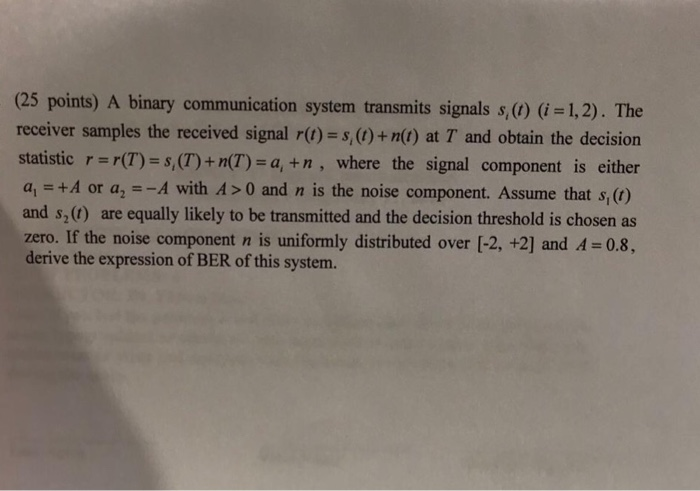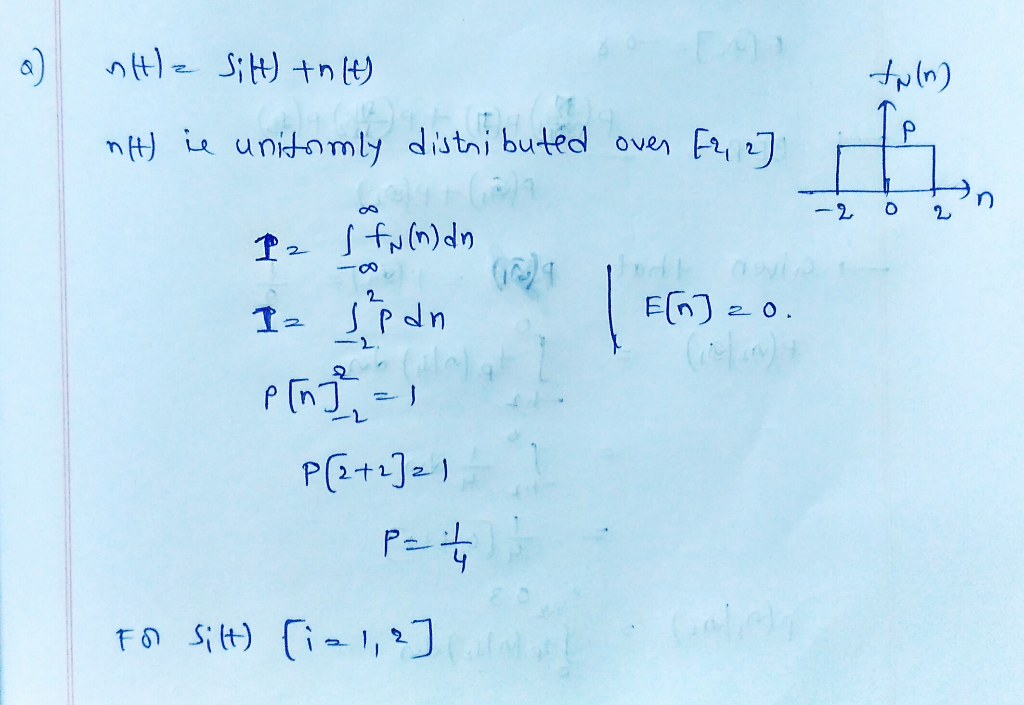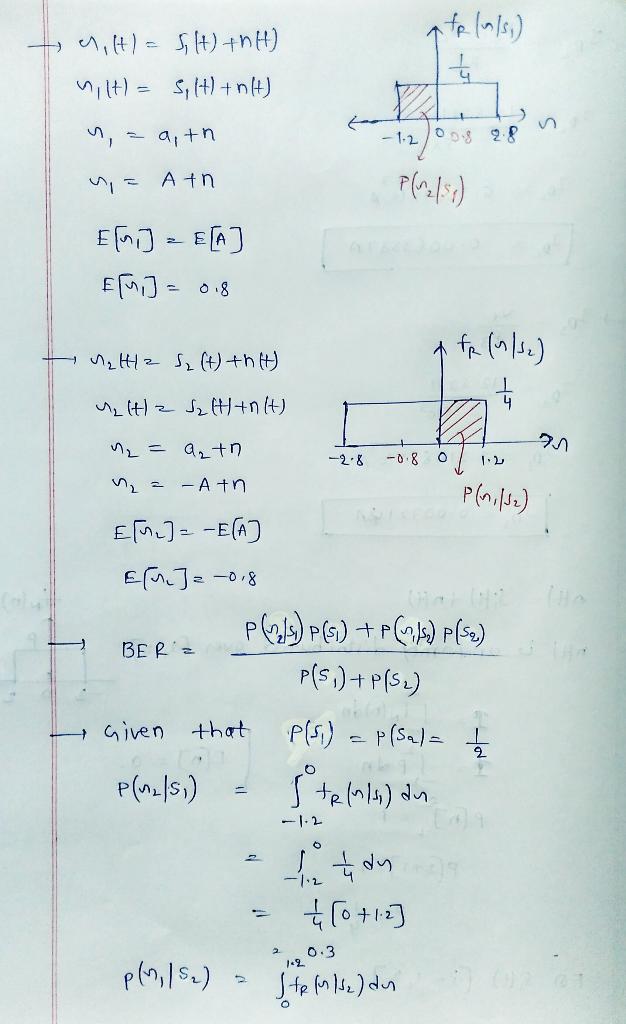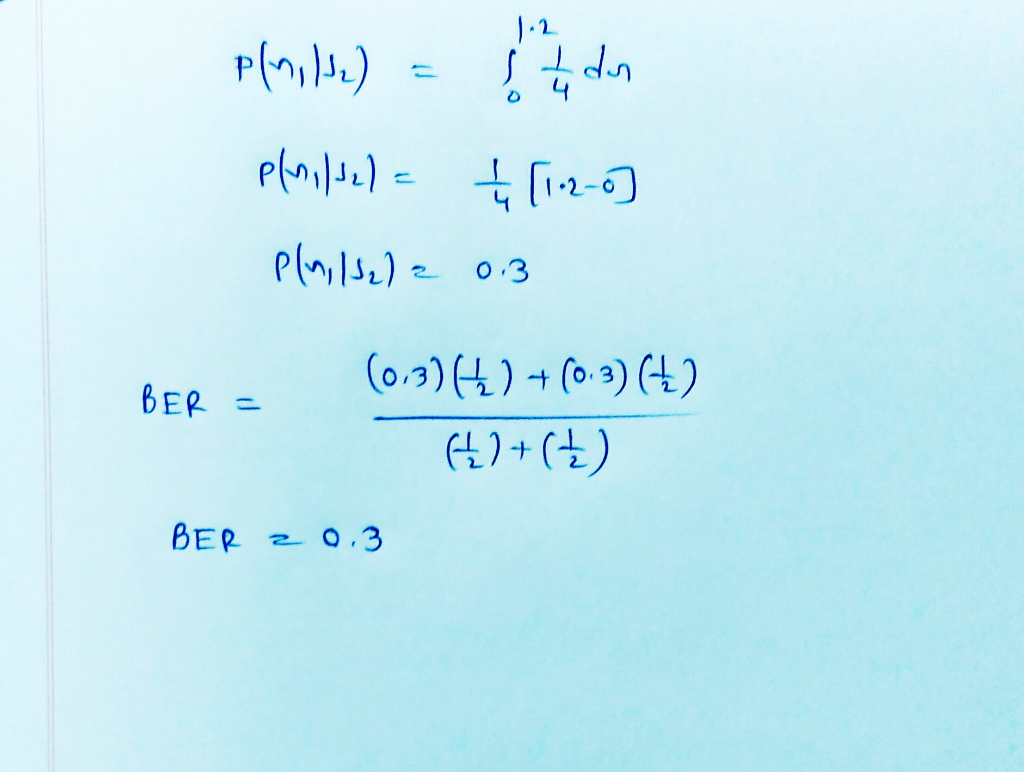#### Earn Coins

Coins can be redeemed for fabulous gifts.

Similar Homework Help Questions
• ### (25 points) A binary communication system transmits signals s,(0) (i1,2). The receiver samples the received signal r(t) s,()+n(t) at T and obtain the decision statistic r-r(T) s,(T)+ n(T)-a, +n,...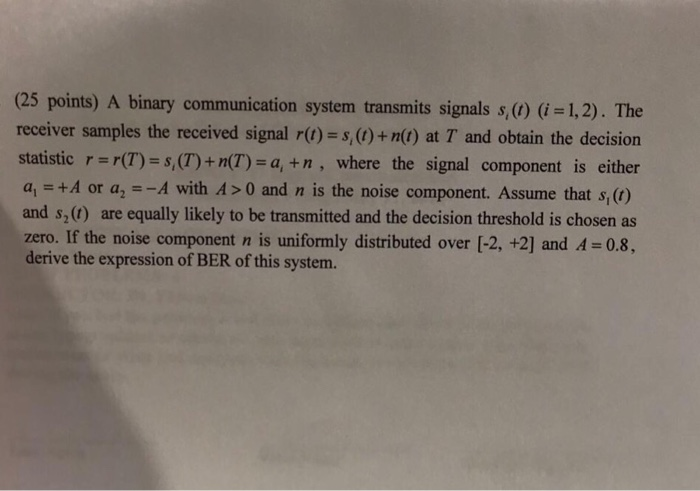(25 points) A binary communication system transmits signals s,(0) (i1,2). The receiver samples the received signal r(t) s,()+n(t) at T and obtain the decision statistic r-r(T) s,(T)+ n(T)-a, +n, where the signal component is either a, = +A or a,--A with A >0 and n is the noise component. Assume that s (t) and s,() are equally likely to be transmitted and the decision threshold is chosen as zero. If the noise component n is uniformly distributed over [-2, +2]...

• ### (25 points) A binary communication system transmits signals s,(0) (i1,2). The receiver samples the received signal r(t) s,()+n(t) at T and obtain the decision statistic r-r(T) s,(T)+ n(T)-a, +n,...(25 points) A binary communication system transmits signals s,(0) (i1,2). The receiver samples the received signal r(t) s,()+n(t) at T and obtain the decision statistic r-r(T) s,(T)+ n(T)-a, +n, where the signal component is either a, = +A or a,--A with A >0 and n is the noise component. Assume that s (t) and s,() are equally likely to be transmitted and the decision threshold is chosen as zero. If the noise component n is uniformly distributed over [-2, +2]...

• ### 3. (30 points) A binary communication system transmits signals s(t) (i 1,2). The receiver samples the received signal r(t) s(t) + n(t) at T and obtain the decision statistic r(T) S (T) n(T) a + n...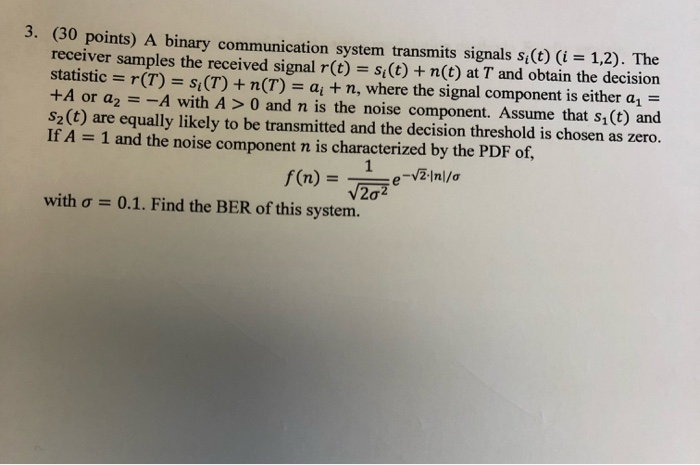3. (30 points) A binary communication system transmits signals s(t) (i 1,2). The receiver samples the received signal r(t) s(t) + n(t) at T and obtain the decision statistic r(T) S (T) n(T) a + n, where the signal component is either an +A or a2-A with A >0 and n is the noise component. Assume that s1 (t) and s2(t) are equally likely to be transmitted and the decision threshold is chosen as zero. If A 1 and the...

• ### 3. (40 points) A binary communication system transmits signals s (0) (i = 1, 2). The...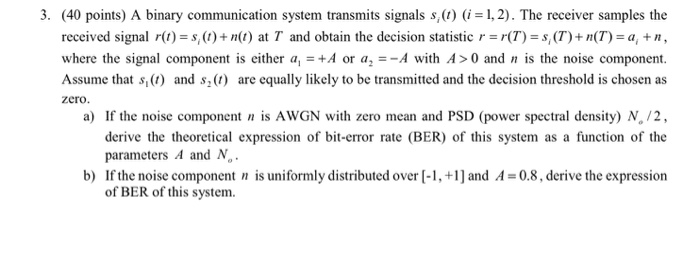3. (40 points) A binary communication system transmits signals s (0) (i = 1, 2). The receiver samples the received signal r(t) = s(t)+ n(t) at T and obtain the decision statistic r =r(T) = S(T) + n(T) = a, un, where the signal component is either a = + A or a, = -A with A >0 and n is the noise component. Assume that s (6) and s(l) are equally likely to be transmitted and the decision threshold...

• ### Problem 4 A base-band digital communication system using binary signals shown in the Figure for transmission...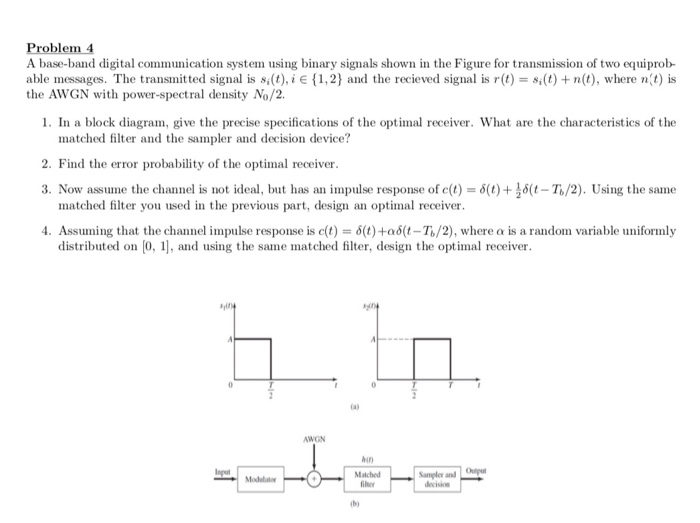Problem 4 A base-band digital communication system using binary signals shown in the Figure for transmission of two equiprob able messages. The transmitted signal is s(t), i e {1,2} and the recieved signal is r(t) s(t)+n(t), where nit) is the AWGN with power-spectral density No/2. 1. In a block diagram, give the precise specifications of the optimal receiver. What are the characteristics of the matched filter and the sampler and decision device? 2. Find the error probability of the optimal...

• ### +00 k-00 The signal z(t) received by a binary communication system can be expressed as z(t)...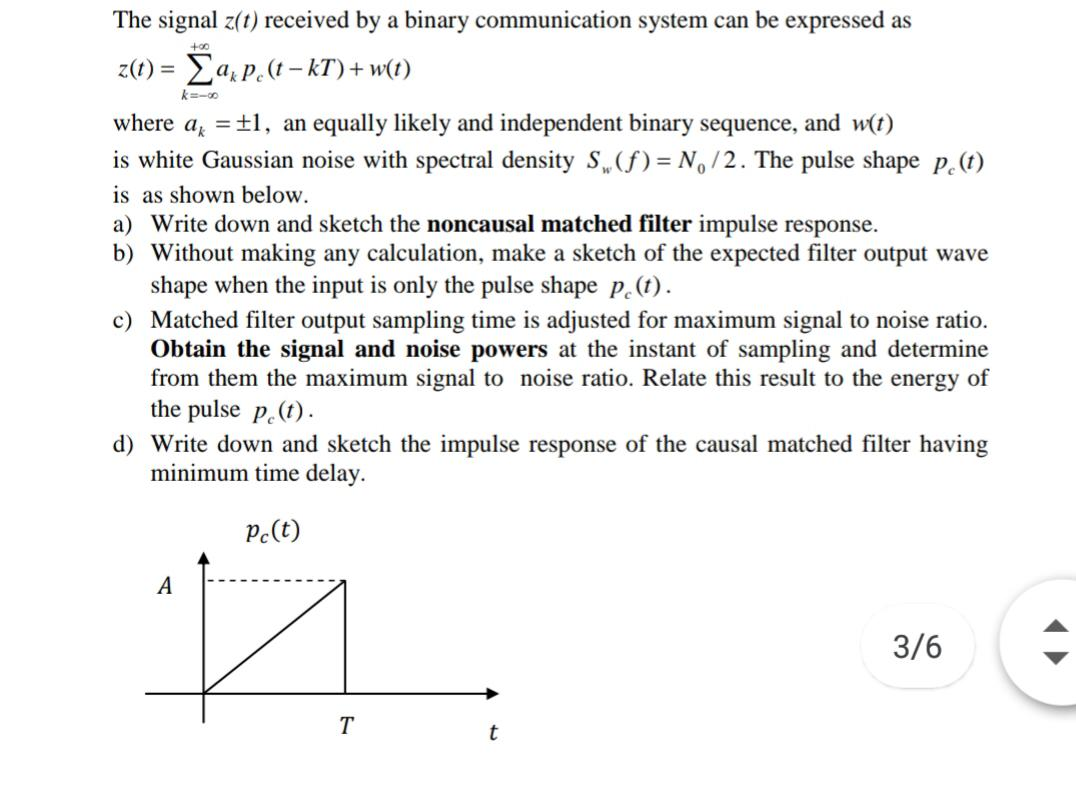+00 k-00 The signal z(t) received by a binary communication system can be expressed as z(t) = axpe(t – kT)+w(t) where ax = £1, an equally likely and independent binary sequence, and w(t) is white Gaussian noise with spectral density S(f)= N, /2. The pulse shape pe(t) is as shown below. a) Write down and sketch the noncausal matched filter impulse response. b) Without making any calculation, make a sketch of the expected filter output wave shape when the input...

• ### 1. Let us consider a digital binary communication system, in which the fol- lowing signal s1(t)...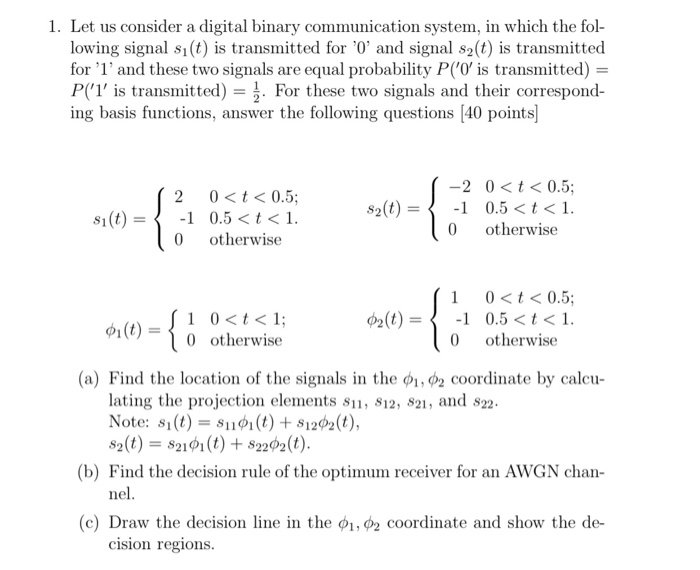1. Let us consider a digital binary communication system, in which the fol- lowing signal s1(t) is transmitted for '0' and signal s2(t) is transmitted for '1' and these two signals are equal probability P('O' is transmitted) P(1' is transmitted). For these two signals and their correspond- ing basis functions, answer the following questions [40 points -2 0t<0.5; 0<t<0.5 -1 0.5 <t< 1 2 s2(t) -1 0.5<t1. s1(t) otherwise 0 otherwise 0 0<t<0.5; -1 0.5 <t1. otherwise 1 10t<1 0...

• ### Consider a matched filter receiver for a binary communication system where a binary '1' is represented by a pulse s() as shown below and a binary 0' is represented by st Consider the...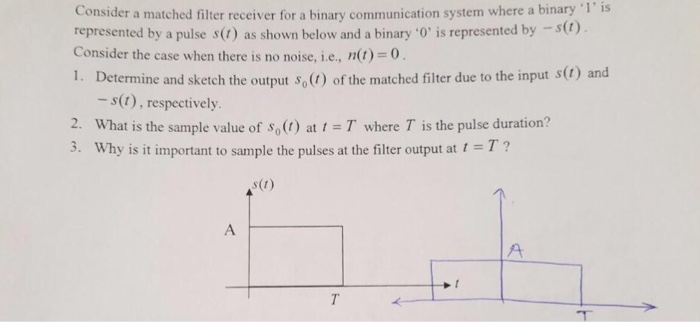Consider a matched filter receiver for a binary communication system where a binary '1' is represented by a pulse s() as shown below and a binary 0' is represented by st Consider the case when there is no noise, i.e, n(t)0 1. Determine and sketch the output s,() of the matched filter due to the input s(1) and - s(t), respectively 2. What is the sample value of so( atT where T is the pulse duration? 3. Why is it...

• ### The input r(t) to a DSBSC receiver is a DSB signal s(t) = A m(t)cos (21fet)...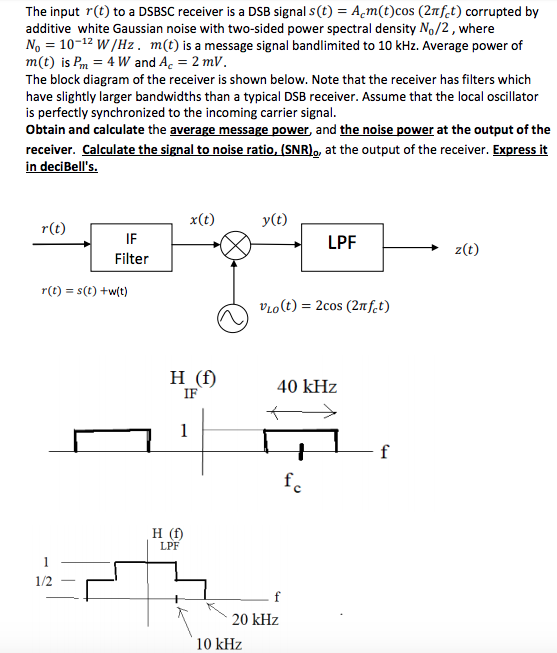The input r(t) to a DSBSC receiver is a DSB signal s(t) = A m(t)cos (21fet) corrupted by additive white Gaussian noise with two-sided power spectral density N,/2, where No = 10-12 W/Hz, m(t) is a message signal bandlimited to 10 kHz. Average power of m(t) is Pm = 4 W and Ac = 2 mV. The block diagram of the receiver is shown below. Note that the receiver has filters which have slightly larger bandwidths than a typical DSB...

• ### Consider a binary communication system that transmits information using the pulse g(t) = A[−u(t) + 2u(t...

Consider a binary communication system that transmits information using the pulse g(t) = A[−u(t) + 2u(t − T /2) − u(t − T )] according to the mapping rule “0′′ → −g(t) “1′′ → +g(t) The “0”s and “1”s are transmitted with equal probability, and the channel is an AWGN channel, with a two-sided noise power spectral density of No/2 watts/Hz. a) Determine and sketch the filter h(t) that is matched to g(t). b) Determine and sketch the overall pulse...